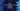# Java compareToIgnoreCase method explanation with an example# Introduction :

compareToIgnoreCase is a public method in the String class of Java. We can use this method to compare two Strings lexicographically and ignoring the case.The provided string is normalized by using _Character.toLowerCase(Character.toUpperCase(character)) _on each character of the String.

## Syntax of compareToIgnoreCase :

The syntax of this method is as below :

``````public int compareToIgnoreCase(String str)
``````

## compareToIgnoreCase Method parameter :

It takes only one parameter str which is the string to compare.

## compareToIgnoreCase Method return type :

compareToIgnoreCase returns one integer value based on the comparison.

Zero: It means that both strings are lexicographically equal. Positive integer: It means that the first string is lexicographically greater than the second string. Negative integer: It means that the first string is lexicographically lesser than the second string.

### Example Program :

Let’s try to understand it more with an example :

``````public class Example {
public static void main(String[] args) {
String str1 = "Hello World";
String str2 = "Hello World";
String str3 = "hello world";
String str4 = "Aello world";

//1
System.out.println("Comparison 1 : "+str1.compareToIgnoreCase(str2));

//2
System.out.println("Comparison 2 : "+str1.compareToIgnoreCase(str3));

//3
System.out.println("Comparison 3 : "+str1.compareToIgnoreCase(str4));

//4
System.out.println("Comparison 4 : "+str3.compareToIgnoreCase(str4));

//5
System.out.println("Comparison 5 : "+str4.compareToIgnoreCase(str3));
}
}
``````

It will print the below output :

``````Comparison 1 : 0
Comparison 2 : 0
Comparison 3 : 7
Comparison 4 : 7
Comparison 5 : -7
``````

### Explanation :

The commented numbers in the above example denote the step numbers below :

1. The first print statement prints_ 0_ as both str1 and str2 are same.

2. This print statement prints 0. str1 and str3 are not same, but if we don’t consider the case of each character, then they are actually lexicographically equal strings.

3. str1 and str4 are not the same. The only difference is between_ ‘H’ and ‘A’._ If we consider the lower case for both, the ASCII value of h is 104 and a is 97. The difference is 7. str1 is greater than str4 lexicographically.

4. Similar to the above step, ASCII difference is 7.

5. It prints_ -7_. The reason is the same as step 3 and step 4.

### Conclusion :

compareToIgnoreCase method makes it easier to compare two strings lexicographically in Java. Try to run the examples above and drop one comment below if you have any queries regarding this tutorial.